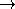## ExtremalPolygonTraits_2advanced### Definition

The concept ExtremalPolygonTraits_2 provides the types and operations needed to compute a maximal k-gon that can be inscribed into a given convex polygon.

### Types

 ExtremalPolygonTraits_2::FT model for FieldNumberType. ExtremalPolygonTraits_2::Point_2 model for Kernel::Point_2. ExtremalPolygonTraits_2::Less_xy_2 model for Kernel::Less_xy_2. ExtremalPolygonTraits_2::Orientation_2 model for Kernel::Orientation_2. ExtremalPolygonTraits_2::Operation AdaptableBinaryFunction class op: Point_2  × Point_2FT. Together with init this operation recursively defines the objective function to maximize. Let p and q be two vertices of a polygon P such that q precedes p in the oriented vertex chain of P starting with vertex root. Then op(p,q) returns the value by which an arbitrary sub-polygon of P with vertices from [root, q] increases when p is added to it. E.g. in the maximum area case this is the area of the triangle (root, q, p).

### Operations

int t.min_k () const returns the minimal k for which a maximal k-gon can be computed. (e.g. in the maximum area case this is three.)

FT t.init ( const Point_2& p, const Point_2& q) const
returns the value of the objective function for a polygon consisting of the two points p and q. (e.g. in the maximum area case this is FT( 0).)

Operation t.operation ( const Point_2& p) const
return Operation where p is the fixed root point.

template < class RandomAccessIterator, class OutputIterator >
OutputIterator
 t.compute_min_k_gon ( RandomAccessIterator points_begin, RandomAccessIterator points_end, FT& max_area, OutputIterator o)
const
writes the points of [points_begin, points_end) forming a min_k()-gon rooted at points_begin of maximal value to o and returns the past-the-end iterator for that sequence (== o + min_k()).

Less_xy_2 t.less_xy_2_object ()
Orientation_2 t.orientation_2_object ()

### Has Models

CGAL::Extremal_polygon_area_traits_2<K>
CGAL::Extremal_polygon_perimeter_traits_2<K>advanced